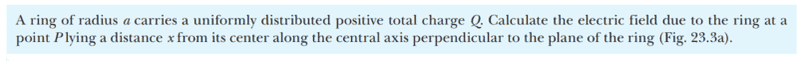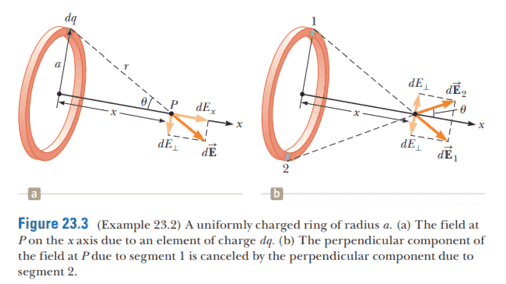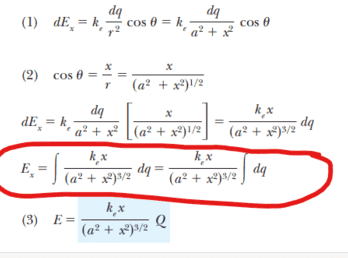# No Limits of Integration for Electric Field Integral?

Callumnc1
Homework Statement:
This is a more concise version of my 'Electric Field of a Uniform Ring of Charge' thread that I posted yesterday and made a typo.
Relevant Equations:
Continuous charge distribution formula
For this problem,The solution is,However, why have they not included limits of integration? I think this is because all the small charge elements dq across the ring add up to Q.

However, how would you solve this problem with limits of integration?

Many thanks!

Homework Helper
Gold Member
You can assume a uniform linear charge density on the ring, ##\lambda=\dfrac{Q}{2\pi a}.## Then an arc element ##ds## at polar angle ##\phi## in the plane of the ring subtends angle ##d\phi## so that ##ds=a d\phi##. The charge on that element is ##dq=\lambda ds=\lambda a d\phi## so that when you integrate going around the ring once, you have $$\int dq=\int_0^{2\pi}\lambda a d\phi=2\pi a\lambda=2\pi a\frac{Q}{2\pi a}=Q$$Does this answer your question?

•Callumnc1
Callumnc1
You can assume a uniform linear charge density on the ring, ##\lambda=\dfrac{Q}{2\pi a}.## Then an arc element ##ds## at polar angle ##\phi## in the plane of the ring subtends angle ##d\phi## so that ##ds=a d\phi##. The charge on that element is ##dq=\lambda ds=\lambda a d\phi## so that when you integrate going around the ring once, you have $$\int dq=\int_0^{2\pi}\lambda a d\phi=2\pi a\lambda=2\pi a\frac{Q}{2\pi a}=Q$$Does this answer your question?

Callumnc1
You can assume a uniform linear charge density on the ring, ##\lambda=\dfrac{Q}{2\pi a}.## Then an arc element ##ds## at polar angle ##\phi## in the plane of the ring subtends angle ##d\phi## so that ##ds=a d\phi##. The charge on that element is ##dq=\lambda ds=\lambda a d\phi## so that when you integrate going around the ring once, you have $$\int dq=\int_0^{2\pi}\lambda a d\phi=2\pi a\lambda=2\pi a\frac{Q}{2\pi a}=Q$$Does this answer your question?
Sorry @kuruman, why are you allowed to use the linear charge density, because doesn't the ring have a width too?

Are you assuming that x >> width, so the width is negligible?

Many thanks!

••